Test: Short Transmission Line

# Test: Short Transmission Line

Test Description

## 10 Questions MCQ Test Power Systems | Test: Short Transmission Line

Test: Short Transmission Line for Electrical Engineering (EE) 2023 is part of Power Systems preparation. The Test: Short Transmission Line questions and answers have been prepared according to the Electrical Engineering (EE) exam syllabus.The Test: Short Transmission Line MCQs are made for Electrical Engineering (EE) 2023 Exam. Find important definitions, questions, notes, meanings, examples, exercises, MCQs and online tests for Test: Short Transmission Line below.
Solutions of Test: Short Transmission Line questions in English are available as part of our Power Systems for Electrical Engineering (EE) & Test: Short Transmission Line solutions in Hindi for Power Systems course. Download more important topics, notes, lectures and mock test series for Electrical Engineering (EE) Exam by signing up for free. Attempt Test: Short Transmission Line | 10 questions in 30 minutes | Mock test for Electrical Engineering (EE) preparation | Free important questions MCQ to study Power Systems for Electrical Engineering (EE) Exam | Download free PDF with solutions
 1 Crore+ students have signed up on EduRev. Have you?
Test: Short Transmission Line - Question 1

### Shunt capacitance is neglected in the analysis of which transmission lines?

Detailed Solution for Test: Short Transmission Line - Question 1

Types of transmission lines:
Depending upon the length of the line, it is divided into three types: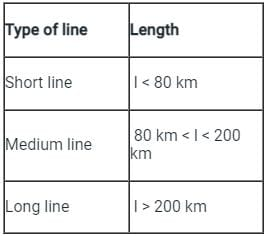Shunt capacitance is neglected in the analysis of short transmission lines. This is because:
The capacitive reactance is given by: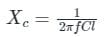Since the value of length (l) is small in the short transmission lines, therefore the reactance becomes very high.
High reactance means an open circuit, hence effect of capacitance is neglected in the short transmission lines.

Test: Short Transmission Line - Question 2

### A short transmission line has impedance value Z. The values of A, D, B and C of the short transmission line are _______, respectively

Detailed Solution for Test: Short Transmission Line - Question 2

Concept: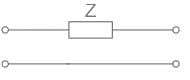The ABCD parameters of the above circuit diagram are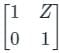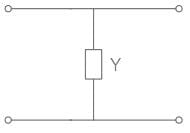The ABCD parameters of the above circuit diagram are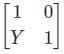When the networks are connected in cascade, their ABCD parameters will get multiplied.
Calculation:
A short transmission line can be modelled as a series impedance
z = R + jωL
The ABCD constants of this series impedance are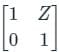A = D = 1, B = z, C = 0

Test: Short Transmission Line - Question 3

### When the length of an overhead transmission line is less than 80 km with an operating voltage upto 20 kV, it is considered to be a/an ___________.

Detailed Solution for Test: Short Transmission Line - Question 3

• A transmission line has three constants R, L, and C distributed uniformly along the whole length of the line.
• The resistance and inductance form the series impedance.
• The capacitance existing between conductors for a 1-phase line or from a conductor to neutral for a 3-phase line forms a shunt path throughout the length of the line. Therefore, capacitance effects introduce complications in transmission line calculations.

Depending upon the manner in which capacitance is taken into account, the overhead transmission lines are classified as:
Short transmission lines:

When the length of an overhead transmission line is up to about 50 km to 80 km and the line voltage is comparatively low (< 20 kV), it is usually considered as a short transmission line. Due to smaller lengths and lower voltage, the capacitance effects are small and hence can be neglected. Therefore, while studying the performance of a short transmission line, only the resistance and inductance of the line are taken into account.

Medium transmission lines:
When the length of an overhead transmission line is about 80-150 km and the line voltage is moderately high (>20 kV < 100 kV), it is considered a medium transmission line. Due to the sufficient length and voltage of the line, the capacitance effects are taken into account. For purposes of calculations, the distributed capacitance of the line is divided and lumped in the form of condensers shunted across the line at one or more points.

Long transmission lines:
When the length of an overhead transmission line is more than 150 km and line voltage is very high (> 100 kV), it is considered a long transmission line. For the treatment of such a line, the line constants are considered uniformly distributed over the whole length of the line and rigorous methods are employed for the solution

Test: Short Transmission Line - Question 4

The insulation strength of an EHV transmission line is mainly governed by

Detailed Solution for Test: Short Transmission Line - Question 4

Switching surges are the governing factor in the design of insulation for EHV and UHV transmission lines.

Origins of switching overvoltage:

• Energization of transmission lines and cables
• Switching of high-voltage reactors
• Switching of transformers that are loaded by a reactor on their tertiary winding
• Switching of a transformer at no load
• Switching at intermediate substations
• Fault initiation and clearing
Test: Short Transmission Line - Question 5

The effective length of a short transmission line is less than __________.

Detailed Solution for Test: Short Transmission Line - Question 5

Short transmission lines:

• The effective length of a short transmission line is less than 80 km.
• When the length of an overhead transmission line is up to about 50 km to 80 km and the line voltage is comparatively low (< 20 kV), it is usually considered as a short transmission line.
• Due to smaller lengths and lower voltage, the capacitance effects are small and hence can be neglected.
• Therefore, while studying the performance of a short transmission line, only the resistance and inductance of the line are taken into account.
Test: Short Transmission Line - Question 6

A single phase overhead transmission line delivers a power of 5500 kW to a load at 11 kV. The receiving end voltage leads the current by 45°. The resistance and the inductive reactance of the transmission line are 10 Ω and 10 Ω respectively. The sending end voltages is

Detailed Solution for Test: Short Transmission Line - Question 6

Concept:
Short Transmission Line:

When the length of the overhead transmission line is up to about 50 km and the line voltage is comparatively up to 20 kV.
Due to smaller lengths and lower voltage, the capacitance effect is small and may be neglected.
Considered a short transmission line of resistance R and Reactance XL over a length.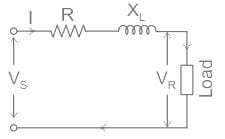Vs is the sending end voltage
VR is the receiving end voltage
cos ϕR is receiving end power factor
and, cos ϕs is sending end power factor
The phasor diagram of the system can be drawn by taking load current as a reference,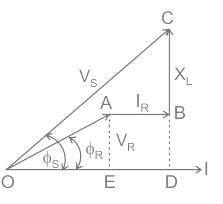From the phasor,
(OC)2 = (OD)2 + (DC)2
(OC)2 = (OE + ED)2 + (DB + BC)2
V2S = (VR cos ϕR + IR)2 + (VR sin ϕR + IXL)2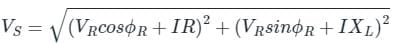Calculation:
Given, P = 5500 kW
VR = 11 kV
R = 10 Ω
XL = 10 Ω
P = VR I cos ϕR
cos ϕR = cos (45°) = 0.707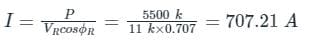From above concept,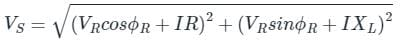sin ϕR = 0.707
(VR cos ϕR + IR) = (11000 × 0.707) + (707.21 × 10) = 14849 volt
(VR sin ϕR + IXL) = (11000 × 0.707) + (707.21 × 10) = 14849 volt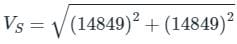⇒ VS = 21 kV

Test: Short Transmission Line - Question 7

Which type of transmission line has length up to 80 km?

Detailed Solution for Test: Short Transmission Line - Question 7

Short Transmission Line:

• When the length of the overhead transmission line is up to about 50 km  - 80 km and the line voltage is comparatively up to 20 kV.
• Due to smaller lengths and lower voltage, the capacitance effect is small and may be neglected.

Considered a short transmission line of resistance R and Reactance XL over a length.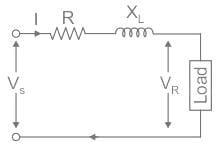Vs is the sending end voltage
VR is the receiving end voltage
cos ϕR is receiving end power factor
and, cos ϕs is sending end power factor
The phasor diagram of the system can be drawn by taking load current as a reference,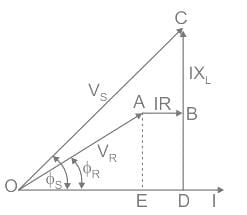From the phasor,
(OC)2 = (OD)2 + (DC)2
(OC)2 = (OE + ED)2 + (DB + BC)2
V2S = (VR cos ϕR + IR)2 + (VR sin ϕR + IXL)2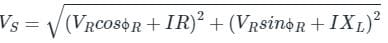Transmission efficiency (η):
The power obtained at the receiving end of a transmission line is generally less than the sending end power due to losses in the line resistance.

The ratio of receiving end power (Power delivered) to the sending end power (Power supplied) of a transmission line is known as the transmission efficiency of the line.

Hence, η = (Power delivered  / Power supplied × 100)

Test: Short Transmission Line - Question 8

A single phase load of 100 KVA is delivered at 2000 V over a transmission line having R = 1.4 Ω and X = 0.8 Ω, when the power factor of load is unity, the voltage at sending end is

Detailed Solution for Test: Short Transmission Line - Question 8

Concept:
In a single phase transmission line
VS = VR + IL∠ϕ Z
Where,
VS = Sending end voltage
VR = Receiving end voltage
IL = Current flowing through the transmission line
Z = Impedance of line
ϕ = Phase angle
Calculation:
Given-
SL = 100 kVA
VR = 2000 V
cos ϕ = 1, ϕ = 0°
Z = R + j X = 1.4 +j 0.8
|IL|= 100 x 103 / 2000 = 50 A
IL ∠ϕ = 50 ∠0°
Now sending end voltage is
VS = 2000 + 50 ∠0° (1.4 +j 0.8)
VS = 2000 + 50(cos0° + sin0°)(1.4 +j 0.8)
VS = 2000 + 70 + j40
VS = 2070 + j40
VS = 2070.40∠1.1°
|V­S| = 2070.40 V

Test: Short Transmission Line - Question 9

Effect of capacitance on performance of the short transmission lines is extremely small due to ________

Detailed Solution for Test: Short Transmission Line - Question 9

Short Transmission Line:
When the length of the overhead transmission line is up to about 50 km and the line voltage is comparatively up to 20 kV.
Due to smaller lengths and lower voltage, the capacitance effect is small and maybe neglected, and hence Shunt admittance too.
Considered a short transmission line of resistance R and Reactance XL over a length.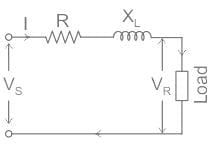Vs is the sending end voltage
VR is the receiving end voltage
cos ϕR is receiving end power factor
and, cos ϕs is sending end power factor
The phasor diagram of the system can be drawn by taking load current as a reference,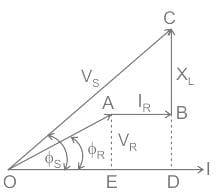From the phasor,
(OC)2 = (OD)2 + (DC)2
(OC)2 = (OE + ED)2 + (DB + BC)2
V2S = (VR cos ϕR + IR)2 + (VR sin ϕR + IXL)2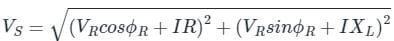Test: Short Transmission Line - Question 10

If the power factor is _____________, the voltage at the sending end is less than that at the receiving end in short line. Hence voltage regulation is negative.

Detailed Solution for Test: Short Transmission Line - Question 10

Voltage regulation: The voltage regulation of transmission line is,
Percentage voltage regulation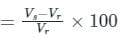Here,
Vs is the sending end voltage
Vr is the receiving end voltage
Voltage Regulation Curve at different Power Factor: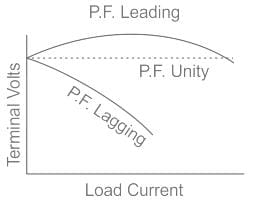From The curve:

• Voltage regulation at the lagging power factor will be more than the voltage regulation at the unity power factor.
• Negative voltage regulation and zero voltage regulation occur at the leading power factor.
• Positive voltage regulation occurs at both unities as well as lagging power factors.

## Power Systems

42 docs|34 tests
 Use Code STAYHOME200 and get INR 200 additional OFF Use Coupon Code
Information about Test: Short Transmission Line Page
In this test you can find the Exam questions for Test: Short Transmission Line solved & explained in the simplest way possible. Besides giving Questions and answers for Test: Short Transmission Line, EduRev gives you an ample number of Online tests for practice

42 docs|34 tests